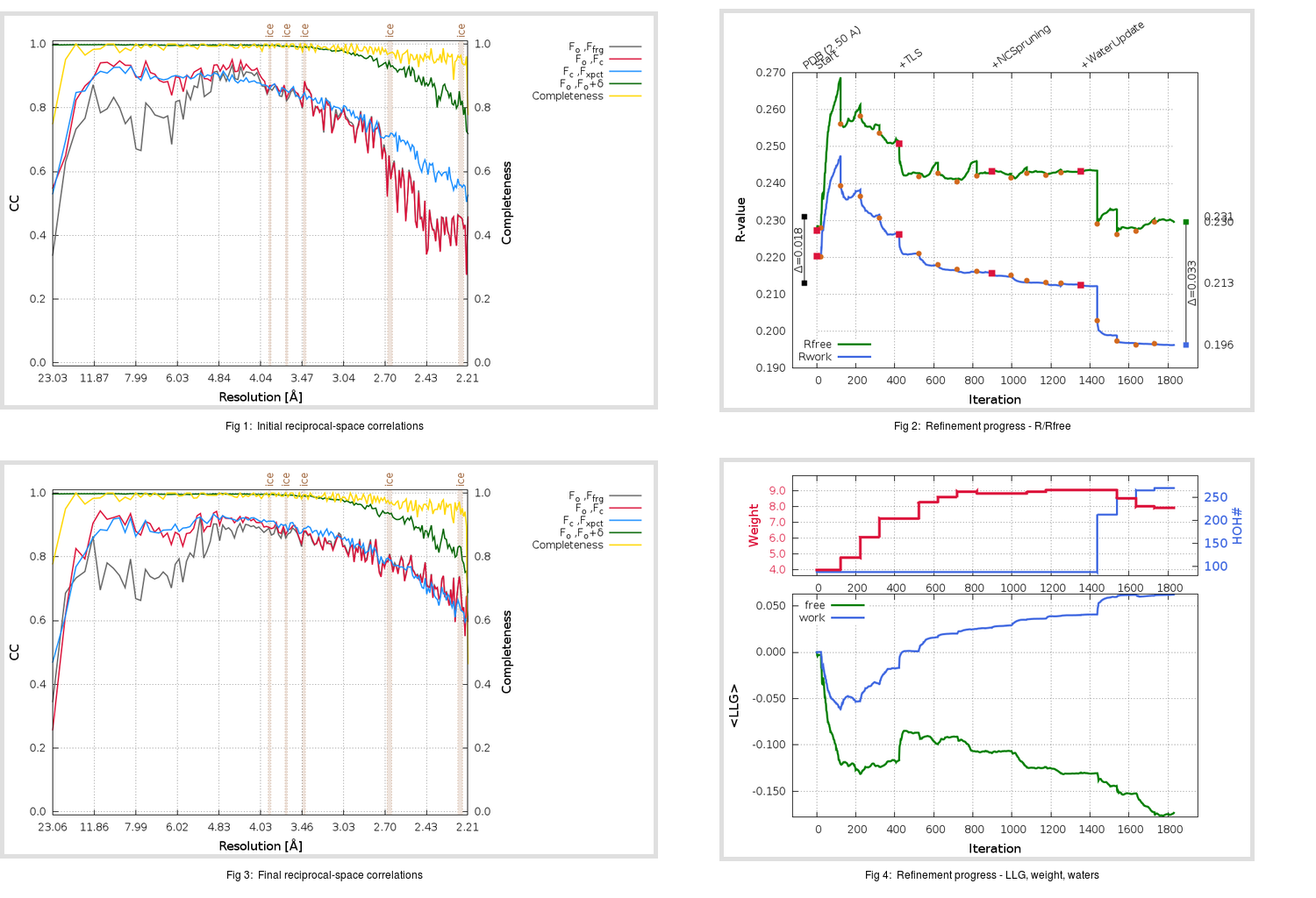Content:

```    Diffraction limits & principal axes of ellipsoid fitted to diffraction cut-off surface:
2.238         0.9092   0.0000   0.4164       0.960 a* + 0.279 c*
2.363         0.0000   1.0000   0.0000       b*
2.095        -0.4164   0.0000   0.9092      -0.313 a* + 0.950 c*
```

## Deposited

` `
 Date deposited Date data collection Resolution R, Rfree 20030814 20030716 2.50 0.2130 0.2310

Molprobity (CCP4 7.0 version) summary:

```Ramachandran outliers =   2.47 %
favored =  89.80 %
Rotamer outliers      =   4.69 %
C-beta deviations     =     0
Clashscore            =  24.48
RMS(bonds)            =   0.0072
RMS(angles)           =   1.98
MolProbity score      =   2.94
Resolution            =   2.50
R-work                =   0.2130
R-free                =   0.2310
```

```Number of waters      =    87

<B> (all atoms) =   39.24 ( sd =   18.63 ) for       4875 non-hydrogen atoms
<B>   (protein) =   39.18 ( sd =   18.75 ) for       4786 non-hydrogen atoms
<B>     (water) =   41.32 ( sd =    6.83 ) for         87 non-hydrogen atoms
<B>    (others) =   88.20 ( sd =   11.84 ) for          2 non-hydrogen atoms

B min/max       (all non-hydrogen atoms) =    4.19 /  125.10
B min/max   (protein non-hydrogen atoms) =    4.19 /  125.10
B min/max     (water non-hydrogen atoms) =   25.11 /   52.44
B min/max     (other non-hydrogen atoms) =   76.36 /  100.04
```

## BUSTER (re-)refinement

` `

Molprobity (CCP4 7.0 version) summary:

```Ramachandran outliers =   0.33 %
favored =  96.05 %
Rotamer outliers      =   5.25 %
C-beta deviations     =     2
Clashscore            =   4.96
RMS(bonds)            =   0.0116
RMS(angles)           =   1.60
MolProbity score      =   2.08
Resolution            =   2.21
R-work                =   0.1964
R-free                =   0.2296
```

```Number of waters      =   270

<B> (all atoms) =   43.76 ( sd =   14.72 ) for       5058 non-hydrogen atoms
<B>   (protein) =   43.74 ( sd =   14.95 ) for       4786 non-hydrogen atoms
<B>     (water) =   43.99 ( sd =    9.61 ) for        270 non-hydrogen atoms
<B>    (others) =   56.22 ( sd =   12.27 ) for          2 non-hydrogen atoms

B min/max       (all non-hydrogen atoms) =   21.85 /  112.37
B min/max   (protein non-hydrogen atoms) =   21.85 /  112.37
B min/max     (water non-hydrogen atoms) =   22.05 /   81.61
B min/max     (other non-hydrogen atoms) =   43.95 /   68.49
```

Refinement progression:Results:

` `
 File Remark 1UK4_aB_refine.01_04_refine.pdb.gz exact refinement commands are in header 1UK4_aB_refine.01_04_refine.mtz.gz including original deposited data and several re-refinement map coefficients 1UK4_aB_refine.01_04_BUSTER_model.cif.gz including any non-standard compound restraints 1UK4_aB_refine.01_04_BUSTER_refln.cif.gz